• 검색 결과가 없습니다.

# Charged particle beam dynamics of space-charge driven resonances and their mitigation in

N/A
N/A
Protected

Share "Charged particle beam dynamics of space-charge driven resonances and their mitigation in"

Copied!
143
0
0
더 보기 ( 페이지)

전체 글

Halo parameters are apparently suppressed for the spinning beams due to the softening effects on the fourth-order particle resonance. Forσz/σx>2, large emittance growth occurs in longitudinal space due to the fourth-order particle resonance and envelope instability (green box).

## Examples of high-intensity accelerators and their applications

In general, the most basic way to use an accelerated beam is to hit a target at the end of the accelerator, producing high-intensity rare-isotope beams (RIBs) or fast and stable particle beams [see Fig. Advanced techniques have been developed to mitigate LLFP and deal with nuclear waste problems, and recently the use of high-intensity beams has been proposed.

## Halo formation in high-intensity accelerators

Now we will derive the space charge force term Fsc generated from the self-generated fields of. 2.6), the resulting space charge fields in transverse and longitudinal planes are expressed by. The alternating gradient of quadrupole magnets is Eext=0, Bextx =Gy,Bexty =Gx, Bextz =0; G≡∂Bextx. 2.10) This also applies to electromagnetic fields. 2.9), the final equations of motion of a single particle under the periodic quadrupole focusing in a real frame (or laboratory frame) become [13, 14].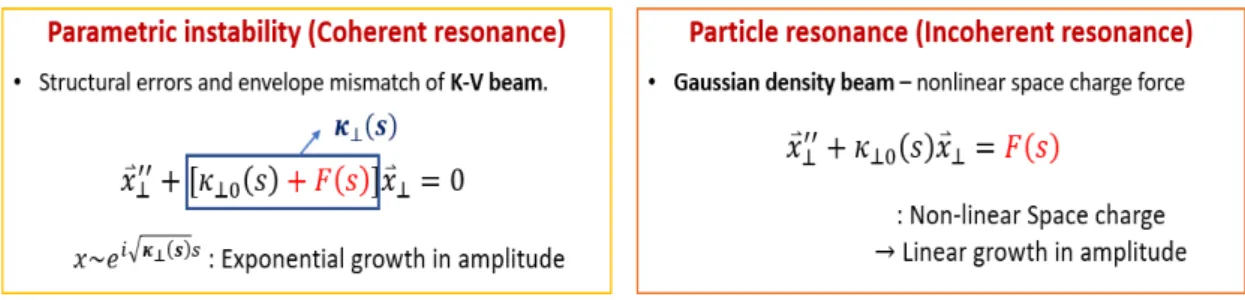Figure 1.3: Simple analysis on the difference between the parametric instability and particle resonances in space-charge dominant beam dynamics.

## Single particle motion in longitudinal space

The orange dot shows the synchronous particle arriving in the same phase of the RF field for a stable energy transfer. If a particle has greater energy than the synchronous particle, it arrives earlier than the synchronous particle and experiences a lower electric field (see the blue dot in Fig. 2.5).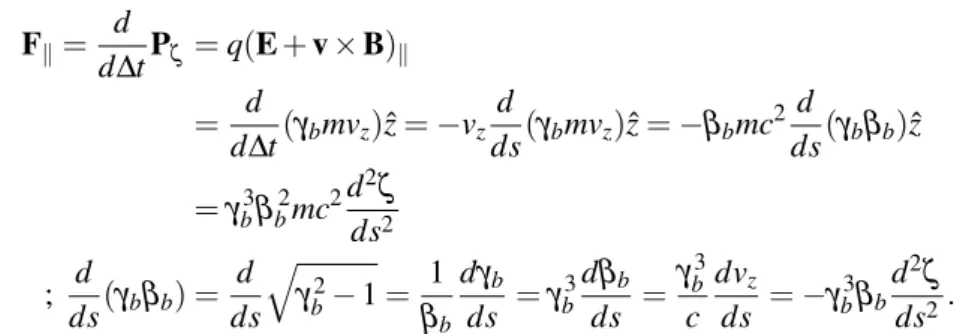Figure 2.4: Longitudinal coordinates of an ellipsoidal bunched beam. φ s denotes the phase of design particle (synchronous particle) which is located at z s and the velocity is v z

## Larmor frame

In the paraxial approximation with p0=γmv∼γbmvz=pz, the normalized mechanical and canonical linear momentum is defined by. Then the derivative of the coordinates in the Larmor frame of Eq. 2.25) and the normalized canonical angular momentum is invariant under the Larmor transform; ˆPθ = xPˆy−yPˆx=XY′−Y X′.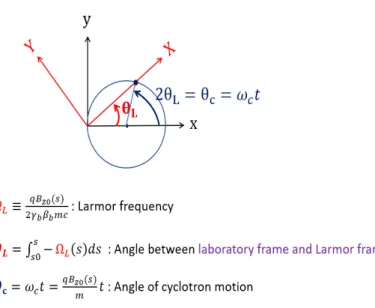Figure 2.6: Larmor transformation of the particle motion from laboratory frame (x,y) to Larmor frame (X,Y)

## K-V beam and envelope equations

Twist parameters are denoted by γxenβx.βxis which is related to the beam size, and γxis which is related to the inclination of the beam in the phase space. Note that the emittance described here corresponds to the total projected emittance, which is 4 times the RMS emittance,εx= 4εx,rms=4p. Twist parameters are denoted by γxenβx, where βxis is related to the beam size, and γxis is related to the inclination of the beam in phase space. and by applying it in Eq. 2.37) with the same mechanism in direction, we can derive the envelope equations of 2D shore beam as [13, 34].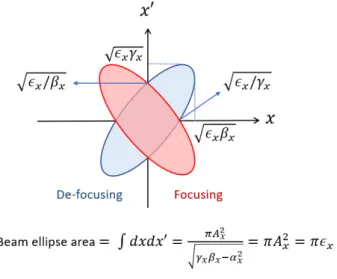Figure 2.8: Phase space (x − x ′ ) distribution in x axis. Beam emittance ε x is the area of the ellipse in the phase space

## Linearized Vlasov-Poisson equation

Beamemittance εxindicates the area of ​​the ellipse in phase space, which includes an entire distribution of particles. The excitation of the parametric instability depends on the state of the external focusing forces at which the zero-current phase advance σ0 is set.

## Space-charge fields

### Space-charge field of Gaussian density beam

From uniform density beam of Eq. 2.29), the RMS beam size can be calculated from the definition of Eq. In this sense, the RMS beam radii for Gaussian density beam can be calculated from envelope equations Eq.

## Equations of motion of 3D bunched beam

### Space-charge field of uniform density beam and 3D bunched envelope equations 23

In addition, the excitation of the envelope instability depends on the extent of the reduced phase advance σ⊥. If σ⊥0>90◦ , envelope instability may occur and the phase advances of the B and Q modes are shifted due to resonances. The excitation of the envelope instability for different zero-current phase advances under the solenoid focusing grid is shown in Fig.

## Parametric nth-order resonance

### Particle-core model

In a real multiparticle system, many single particles form a beam envelope, and their deviations change the envelope radius or beam emittance, which is the average value of the particle distribution. The single-particle wavenumber is half the envelope-mode oscillation wavenumber, kparticle=kmode/2. When the test particles are within the boundary of the beam envelope, they experience the linear space charge force, which is dependent on the beam size.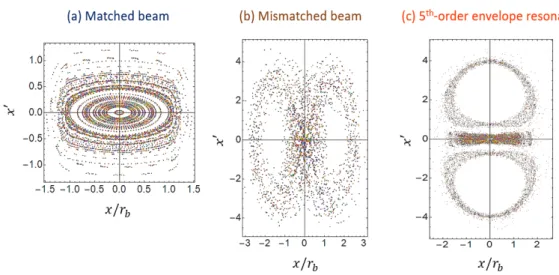Figure 3.9: Particle-core model on the normalized phase space (x/x r b − x ′ ) of (a) matched beam, (b) mismatched beam, and (c) 5th-order envelope resonance

## Self-consistent parametric mode instability

### Analytical interpretation of the fourth-order particle resonance

The four separate islands around the four fixed points on the phase space indicate 4σ =360◦ fourth-order particle resonance. The four islands around the four fixed points on the phase space indicate the 4σ=360◦ fourth-order particle resonance. Furthermore, a detailed particle path of the fourth-order particle resonance is plotted, where the initial state ofr(0)/σr=2.52.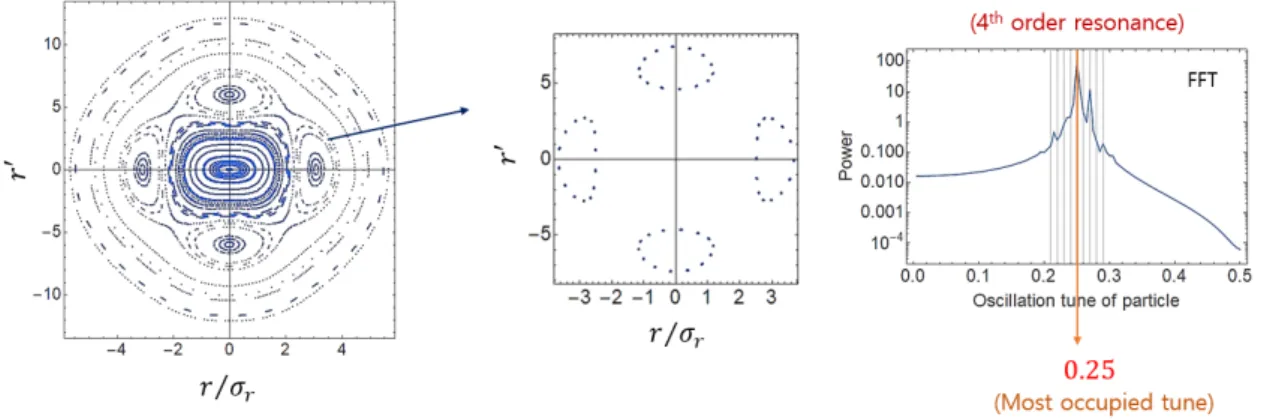Figure 4.1: Poincar´e-section plot of test particles of Gaussian distribution with initially well-matched beam

### Multi-particle simulation of the fourth-order particle resonance

The fourth-order particle resonance 4σ=360◦ is preserved for more than 200 periods and the envelope instability is not ultimately excited. However, the internal principles of fourth-order particle resonance and envelope instability are distinctly different. This means that although the fourth-order particle resonance is manifested, the envelope instability is not induced.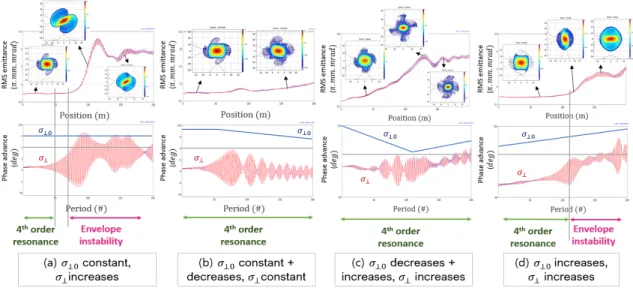Figure 4.3: Numerical simulation results of the change of RMS emittance, phase advances, and (x − x ′ ) phase space plots for 4 different cases along the solenoid focusing linacs

## Introduction of spinning beam

### Equations of motion with non-zero canonical angular momentum

However, for the Gaussian density beam, the non-linear space charge fields make the coupling between xandy when the initial canonical angular momentum is not zero even in the Larmor frame. A particle motion in the real frame and the Larmor frame can be expressed by r and the angle with respect to the x-axis. Also, we can express a particle motion in the Larmor frame by X =r·cosθr andY =r·sinθr, where θr=θ−θL=Rz0z Prˆθ22ds+θ0 is the rotational angle of a particle in the Larmor frame.

### Generation of spinning beam in multiparticle simulation

The angles and particle position on the real frame(x,y) and Larmor frame(X,Y) are shown in Fig. showed. A spin beam has a non-zero mean canonical angular momentum and exhibits rigid rotor rotation about the beam propagation axis. The technology of beam stripping for generating the rotating beam is based on several well-established experiments [65, 66].

## Mitigation effect of spinning beam on the fourth-order particle resonance

### Analytical interpretation of spinning effect

First, we verify a simplified theoretical model for the fourth-order particle resonance state of spin beams that have non-zero canonical angular momentum. When ˆPθ =0, the nonlinear space-charge term in Eq. 6.3) produces an octupole driving 4:1 resonance that excites the 4σ=360◦ fourth-order particle resonance. Since the particle motion in a cylindrical coordinate is fully equivalent to that in Larmor frame, we choose the Larmor frame for the analysis of detuning effect on the fourth-order particle resonance.

### Particle-core model of spinning beam

6.7 (a) and the envelope instability induced after the fourth-order particle resonance after long periods. The lower graph shows the relative emission growth after 200 grating periods, during which the envelope instability is induced after the fourth-order particle resonance. 7.4 (a) and (b), the stop bands for envelope instability and the emission growth patterns are the same in the longitudinal melody depression space, which is independent of the initial RMS emission ratio [see Fig.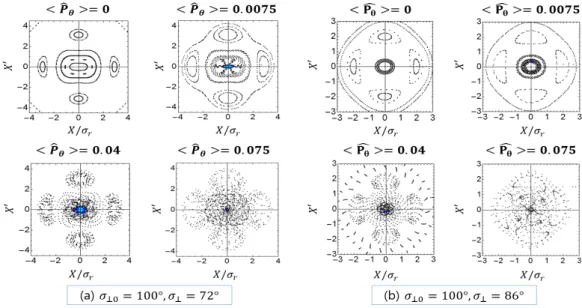Figure 6.4 shows Poincar´e-section plots in real frame when σ ⊥0 = 100 ◦ and σ ⊥ = 72 ◦ of solenoid focusing channel, for (a) ⟨ P ˆ θ ⟩ = 0 non-spinning beam and (b) ⟨ P ˆ θ ⟩ = 0.2 spinning beam

## Fast acceleration in linear accelerators

The initial longitudinal phase advances are σz0=100◦andσz=77◦, which is within the fourth order particle resonance stop band. The fourth-order particle resonance manifests itself during 40 periods and the envelope instability occurs during 100 periods. While the space charge-driven fourth-order particle resonance is clearly observed in cell 10 in Fig.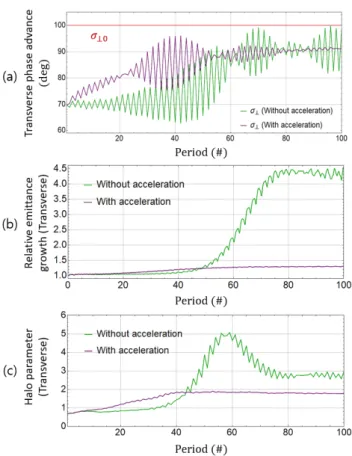Figure 7.7: (a) Transverse phase advances, (b) relative emittance growth, and (c) halo parameter with and without acceleration under the solenoid focusing lattice

## Spinning effect on the fourth-order particle resonance of 3D bunched beam

• Spinning effect in transverse space without acceleration
• Spinning effect in transverse space with acceleration
• Spinning effect in longitudinal space with acceleration
• A parametric study of spinning beam
• Another issues of spinning beam effect
• Design of experiment for applying spinning beam in linear accelerator

The fourth-order resonance is damped for the rotating beam [see red lines in (b) and (c) and plots in (f)]. Although the average canonical angular momentum is not conserved under the quadrupole grating, the effects of beam rotation on the fourth-order resonance are still valid. 7.19 (b), the halo parameters are clearly suppressed for rotating beams due to damping effects on the fourth-order particle resonance.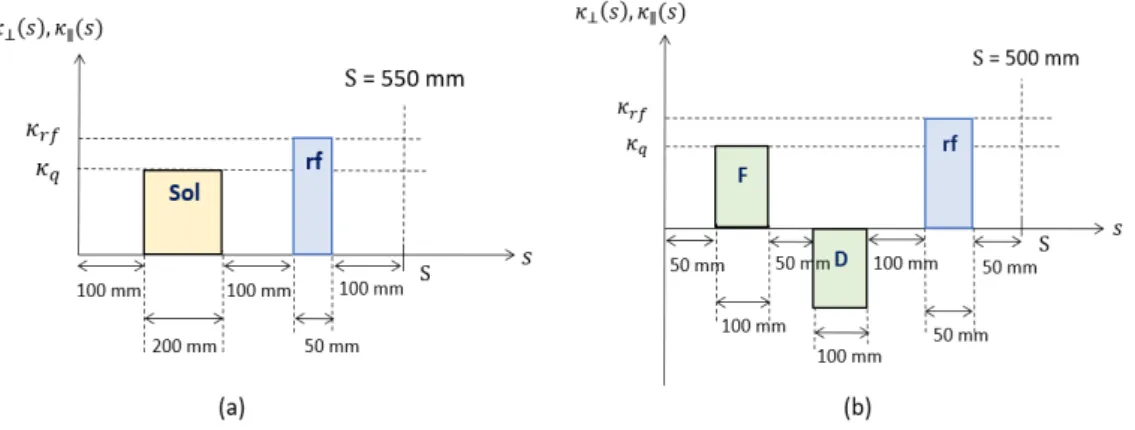Figure 7.10: Periodic focusing lattice for 3D multiparticle simulations without acceleration

## Emittance exchange in 3D bunched beam

Forσz/σx>2 large emittance growth occurs in longitudinal space due to the fourth-order particle resonance and envelope instability (green box). On the other hand, for longitudinal plane (red line), the fourth-order resonance affects more than the effects of the emittance exchange with x-direction. As the transverse emittance increases due to the emittance exchange with metz direction as shown in the blue box of Fig.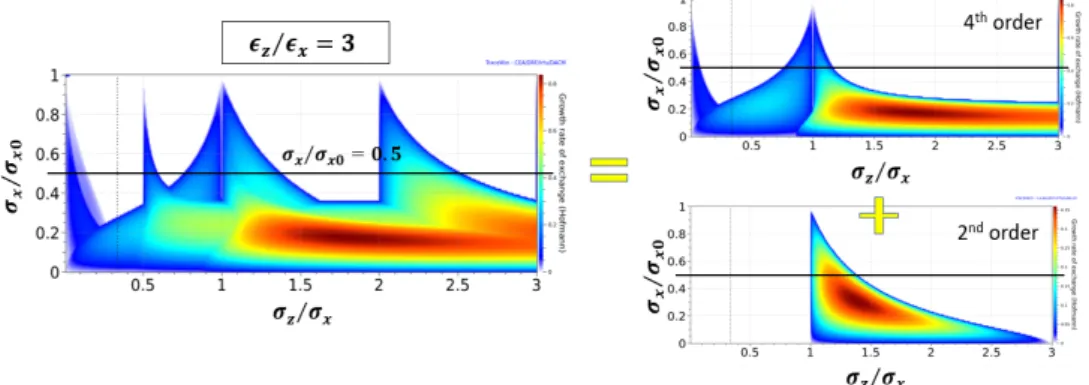Figure 7.21: Hofmann chart from TraceWin simulation code when the initial emittance ratio is ε z /ε x = 3 as a function of tune depression ratios σ x /σ x0 and σ z /σ x

## Machine Protection System (MPS) requirements

### Yield time

This can only happen when the beam immediately hits the gate valve or bellows. right). For example, only when objects (eg, gate valve) are in the beam line or the beam immediately hits the bellows. However, in most cases, the collision angle is expected to be quite small (<15◦), so the response time (~35µs) would be reasonable to protect the beamline components from catastrophic situations.

### Melting time

This is because the maximum value of Ravg corresponds to the higher incident energy of the uranium beam. For angles of incidence greater than 60 degrees, beamline components can be damaged in less than 20 µs, which is beyond the capabilities of the RISP MPS. A 90 degree angle jet injection into the tubing material is the worst case scenario resulting in maximum damage to the components.

MPS response time

## MCNPX simulation studies for beam loss induced radiations

### MCNPX simulation results of beam loss-induced radiation

We then plot dose maps showing the spatial distribution of the beam loss-induced radiation, providing a visual insight into the radiation patterns around the structures. 8.11 (c) and (d) show gamma and neutron radiation patterns from 1 W point (fast) loss of the uranium beam. For the fast loss case, a 1 W point source hits a point on the inner surface of the nozzle (shown by a red dot) with a sweep angle of 10 mrad.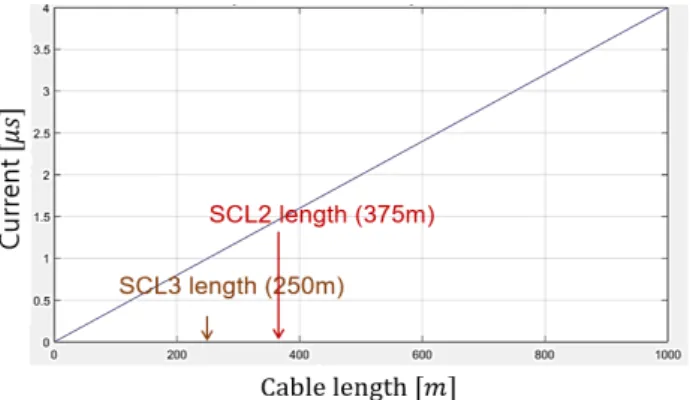Figure 8.6: Signal transfer time as a function of cable length. The LMR-400 signal cable is expected to be used in the RAON BLM system.

The label 1(2) in the bars name indicates that the detector1(2) is used to calculate the dose levels. Furthermore, the energy spectra of radiation loss induced radiation must be distinguished from the background radiation, which is not generated from the radiation loss itself. The label 1(2) in the bars name indicates that the detector1(2) is used to calculate the dose levels.

## Beam Loss Monitors (BLMs)

Since the sensitivity of the IC to the radiation dose resulting from proton or uranium beam losses is too low (only up to ~600 nC/rad), we also consider the use of a proportional counter (PC), which has a much higher sensitivity than that of the IC. The computer creates a larger number of ions and electrons in the chamber, captures more electrons in the anode and increases the intensity of the detected signal by applying a higher voltage than in the case of an IC. Based on the MCNPX simulation results and the characteristics of the detectors, we calculate the expected current signals in the proportional counter (PC) for gamma (see Fig. 8.16) and the plastic detector (PD) for neutrons (see Fig. 8.17).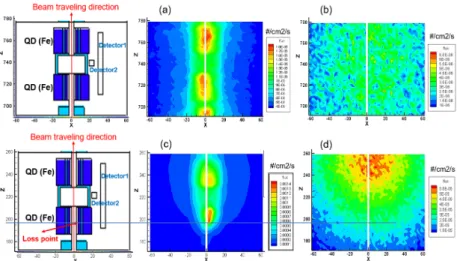Figure 8.11: Neutron and gamma dose maps outside the warm sections induced by beam losses of 200 MeV/u uranium beam

## Configuration of the RAON BLM system

### Final layout of RAON BLM system

Bukh, "Stability properties of the transverse sheath equations describing intense ion beam transport," Physical Review Special Topics-Accelerators and Beams, vol. Maieret al., "Experimental evidence of the 90 stop band in gsi unilac," Physical review letters, vol. Hwang , “Characteristics of fourth-order resonance in high-intensity linear accelerators,” Physics of Plasmas, vol.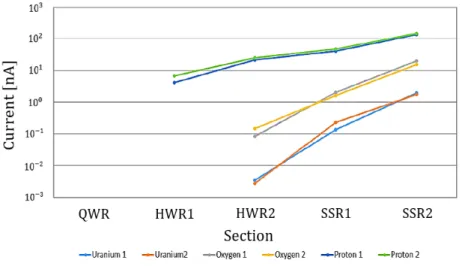Figure 8.16: Proportional counter (PC) current signal for gamma detection. Expected current signal in the beam loss detectors calculated from the radiation levels in Fig

수치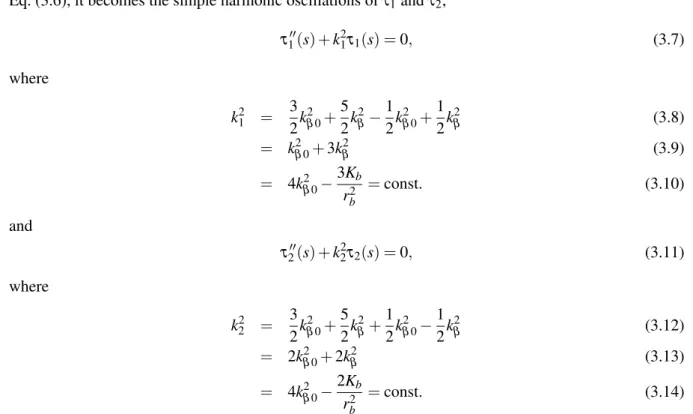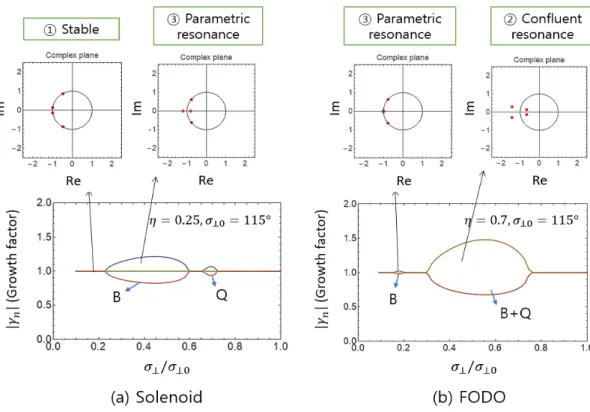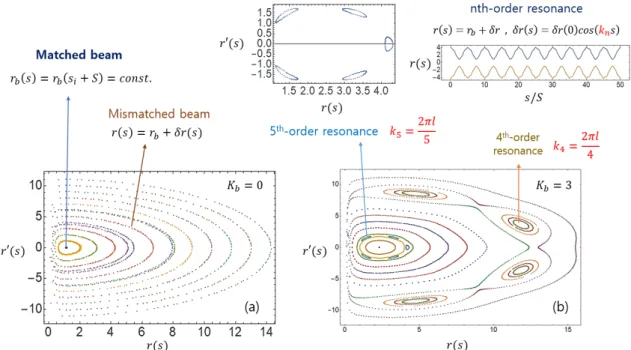+7

참조

관련 문서

High tensile steel with yield strength up to 780 MPa and ultra-high strength concrete with compressive cylinder strength up to 180 MPa were used to construct the test

Keywords: Aspirin exacerbated respiratory disease (AERD), Asthma, Mutual information (MI), Information gain (IG), Genome-wide association study (GWAS), Epistasis,

따라서 본 연구는 요양병원 간호사들의 업무적 특성에 따른 윤리적 갈등 경험 의 본질을 이해하기 위해 현상학적 질적 연구를 하고자 한다 현상학적 질적 연.. 따라

follows that (neglecting the small difference in the electron binding

(i) Nuclear forensic capabilities as an element of a national response plan (Technical Session 2A): The session focused on the progress of Member States in using the

The means of implementation (MOI) - finance, technology development and transfer and capacity building - should be an integral part of the 2015 agreement. All developed country

19 September Saint Kitts and Nevis 19 September. Saint Lucia

Transport Canada’s Airports Operations and Maintenance Subsidy Program (The Program) provides financial support to remote airports that are identified in the National Airports Policy

AMRO also released its ASEAN+3 Regional Economic Outlook (AREO 2020) report, and it reduced the ASEAN+3 growth projection to just two percent in 2020 and then improve to 5.5 percent

언어 선택하기

웹사이트는 선택한 언어로 번역됩니다.

기타 언어